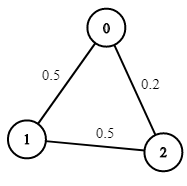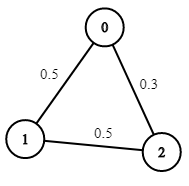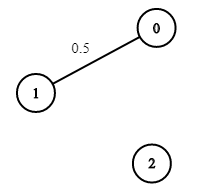# Path with Maximum Probability

### 描述​

You are given an undirected weighted graph of n nodes (0-indexed), represented by an edge list where edges[i] = [a, b] is an undirected edge connecting the nodes a and b with a probability of success of traversing that edge succProb[i].

Given two nodes start and end, find the path with the maximum probability of success to go from start to end and return its success probability.

If there is no path from start to end, return 0. Your answer will be accepted if it differs from the correct answer by at most 1e-5.

Example 1:Input: n = 3, edges = [[0,1],[1,2],[0,2]], succProb = [0.5,0.5,0.2], start = 0, end = 2
Output: 0.25000
Explanation: There are two paths from start to end, one having a probability of success = 0.2 and the other has 0.5 * 0.5 = 0.25.

Example 2:Input: n = 3, edges = [[0,1],[1,2],[0,2]], succProb = [0.5,0.5,0.3], start = 0, end = 2
Output: 0.30000

Example 3:Input: n = 3, edges = [[0,1]], succProb = [0.5], start = 0, end = 2
Output: 0.00000
Explanation: There is no path between 0 and 2.

Constraints:

• $2 <= n <= 10^4$
• 0 <= start, end < n
• start != end
• 0 <= a, b < n
• a != b
• 0 <= succProb.length == edges.length <= $2*10^4$
• 0 <= succProb[i] <= 1
• There is at most one edge between every two nodes.

### 代码​

// Path with Maximum Probability// Dijkstra// Time Complexity: O(ElogN), Space Complexity: O(N + E)class Solution {    public double maxProbability(int n, int[][] edges, double[] succProb, int start, int end) {        // adjacency list, map<vertex_id, map<vertex_id, weight>>        Map<Integer, Map<Integer, Double>> graph = new HashMap<>();        for (int i = 0; i < edges.length; i++) {            int[] edge = edges[i];            double w = -Math.log(succProb[i]);            // Undirected            graph.putIfAbsent(edge, new HashMap<>());            graph.get(edge).put(edge, w);            graph.putIfAbsent(edge, new HashMap<>());            graph.get(edge).put(edge, w);        }        Map<Integer, Double> dist = dijkstra(graph, start);        return dist.containsKey(end) ? Math.exp(-dist.get(end)) : 0;    }    /** Standard Dijkstra algorithm.     *     @param graph Adjacency list, map<vertex_id, map<vertex_id, weight>>.     @param start The starting vertex ID.     @return dist, map<vertex_id, distance>.     */    private static Map<Integer, Double> dijkstra(Map<Integer, Map<Integer, Double>> graph, int start) {        // map<vertex_id, distance>        Map<Integer, Double> dist = new HashMap<>();        // vertex_id -> father_vertex_id        Map<Integer, Integer> father = new HashMap<>();        // pair<distance, vertex_id>, min heap, sorted by distance from start to vertex_id        Queue<Pair<Double, Integer>> pq = new PriorityQueue<>((a, b) -> Double.compare(a.getKey(), b.getKey()));        // from start to start itself        pq.offer(new Pair(0.0, start));        dist.put(start, 0.0);        while(!pq.isEmpty()){            final int u = pq.poll().getValue();            if (!graph.containsKey(u)) continue; // leaf node            for(int v : graph.get(u).keySet()){                final double w = graph.get(u).get(v);                if (!dist.containsKey(v) || dist.get(u)+ w < dist.get(v)) {                    final double shorter = dist.get(u)+ w;                    dist.put(v, shorter);                    father.put(v, u);                    pq.offer(new Pair(shorter, v));                }            }        }        return dist;    }}